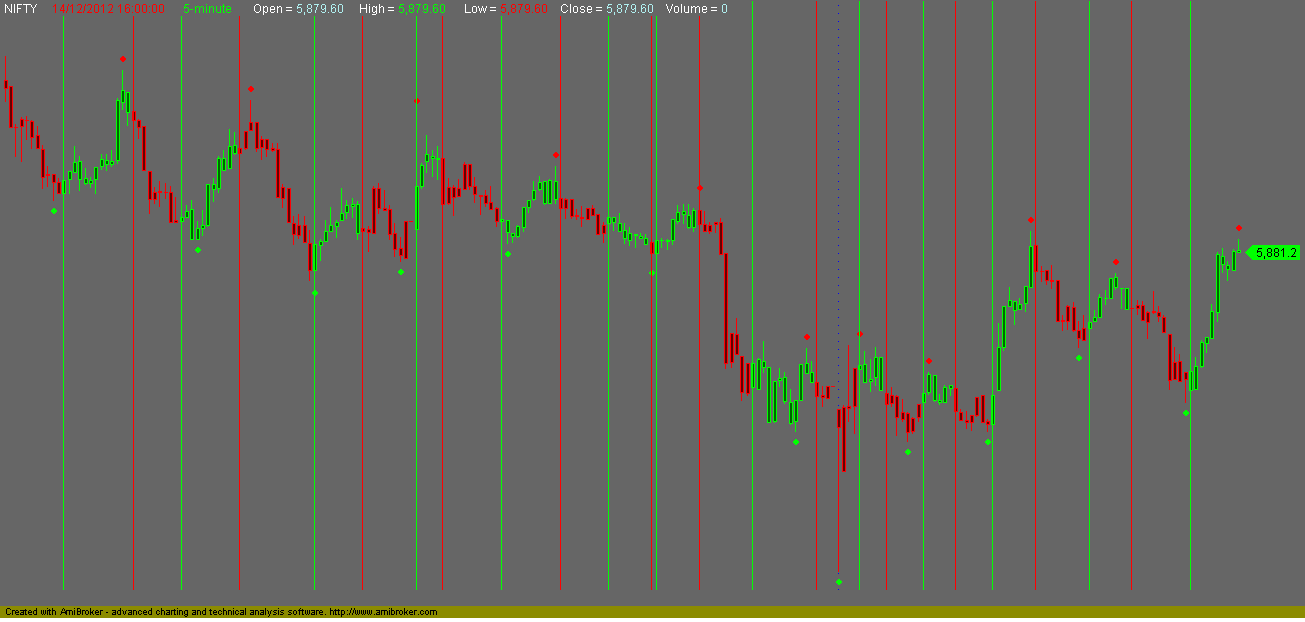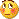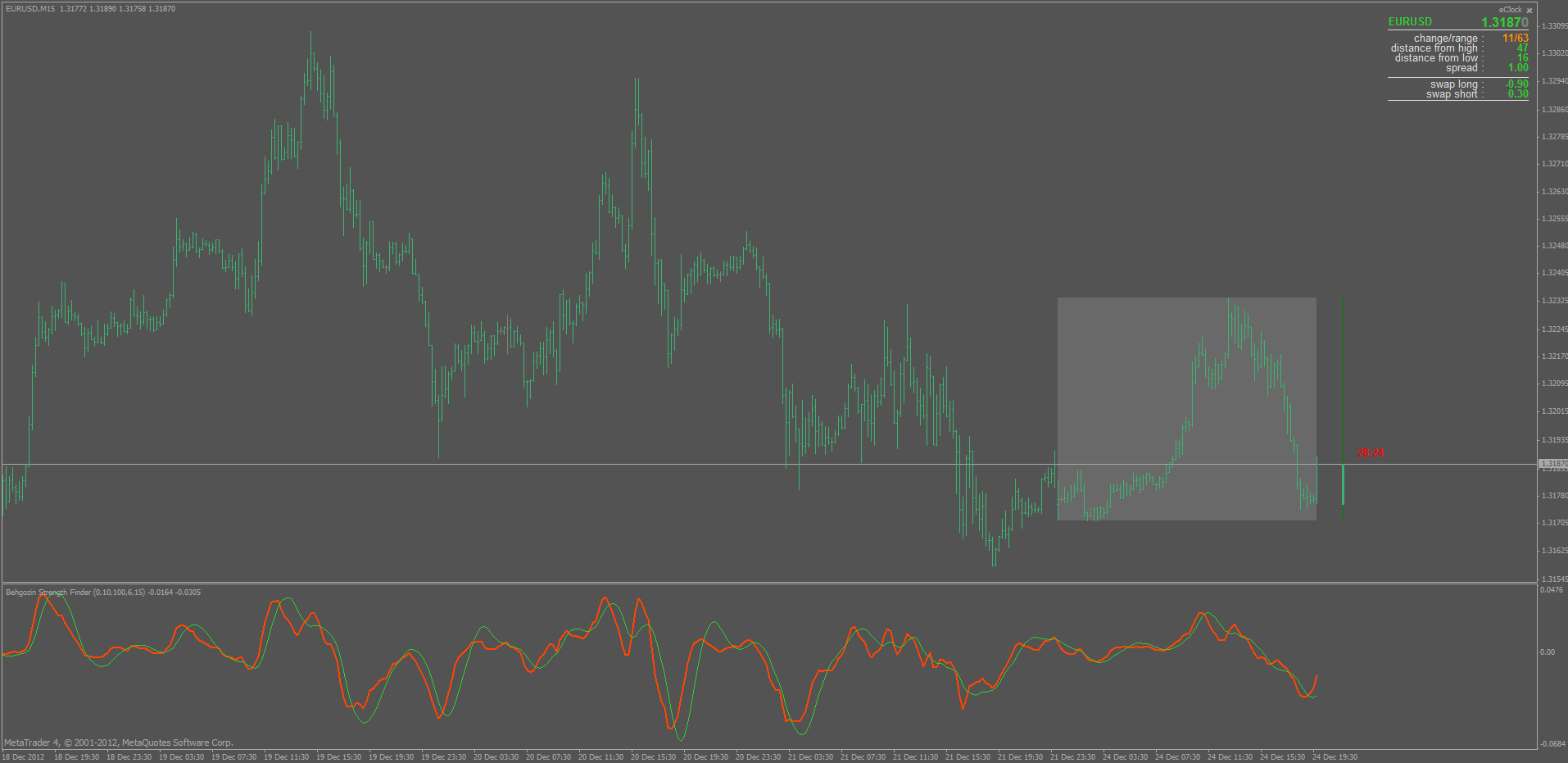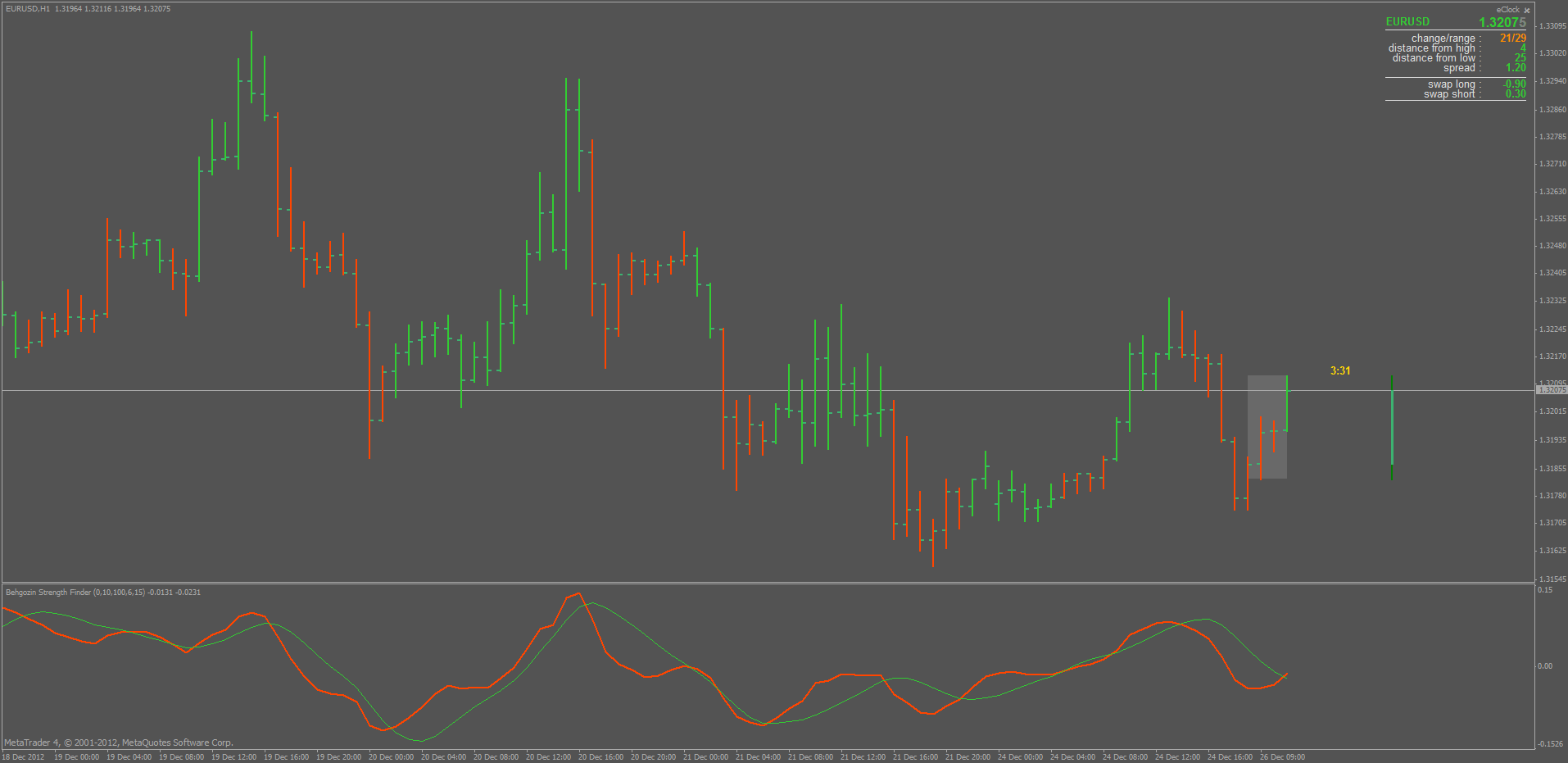# Indicators with alerts/signal - page 455154346

Volatility quality - zero line with alerts posted here : https://www.mql5.com/en/forum/general12

Help modifying EA

mrtools:

Hello MrTools,

Can you point me in the right direction as to who can help with an EA modification?

Many thanks.

Pip Singh59

few days back you converted amibroker indicator name "Behgozinstrengthfinder" to mt4, you remember?

give buy/sell signal when it cross zero value.

though i am very novice in technical, i have some stupid idea on it. i take behgozin and its LinearRegression-LeastSquaresMovingAverage on same window and marked the crossover, then paint it on bar color.

here is the amibroker code about it..

_SECTION_BEGIN("Behgozin");

CLN= Ref(C,0);

Beh=((CLN - EMA(CLN, 10)) / EMA(CLN, 10)) * 100;

V2 = HHV(Beh,100);

V3 = LLV(Beh,100);

Beh1= WMA(Beh*(V2-V3),6);

_SECTION_END();

_SECTION_BEGIN("Linear Regression");

P = Beh1;

Periods = Param("Periods", 15, 2, 300, 1, 10 );

_SECTION_END();

_SECTION_BEGIN("barcolor");

SetBarFillColor( IIf(Beh1 > LinearReg( P, Periods ) ,ColorRGB( 0, 100, 0 ),ColorRGB( 100,0,0 )));

SetChartOptions(0,chartShowDates);

SetChartBkColor( ParamColor("Color", ColorRGB( 105, 105, 105 ) ) );

GraphXSpace=Param("GraphXSpace",10,-100,100,1);

Plot(C,"Close",IIf(Beh1 > LinearReg( P, Periods ) ,ColorRGB( 0,255, 0 ),ColorRGB(255,0,5 )),styleCandle);

_SECTION_END();above is the picture how its look like. sometime it work as "too good to be true" yet not repainted but sometime fail badly.

guru,

please can you make the code for mt4?and is there any way to improve it??

Files:
86426605.png 12 kb5

hi

can anybdy recommend me good EA or a set/system of indicators which can help me give signals/entry exit points ? thankz3

Hi,mrtools and other programmer.

But arrow signal appear in sub window.

I want to appear this arrow signal on main window.

Thanks.

Files:31

elam forex

elam_forex_system.rar if she could do this to someone that works thanks

Files:109

debashis:
few days back you converted amibroker indicator name "Behgozinstrengthfinder" to mt4, you remember?

give buy/sell signal when it cross zero value.

though i am very novice in technical, i have some stupid idea on it. i take behgozin and its LinearRegression-LeastSquaresMovingAverage on same window and marked the crossover, then paint it on bar color.

here is the amibroker code about it..

_SECTION_BEGIN("Behgozin");

CLN= Ref(C,0);

Beh=((CLN - EMA(CLN, 10)) / EMA(CLN, 10)) * 100;

V2 = HHV(Beh,100);

V3 = LLV(Beh,100);

Beh1= WMA(Beh*(V2-V3),6);

_SECTION_END();

_SECTION_BEGIN("Linear Regression");

P = Beh1;

Periods = Param("Periods", 15, 2, 300, 1, 10 );

_SECTION_END();

_SECTION_BEGIN("barcolor");

SetBarFillColor( IIf(Beh1 > LinearReg( P, Periods ) ,ColorRGB( 0, 100, 0 ),ColorRGB( 100,0,0 )));

SetChartOptions(0,chartShowDates);

SetChartBkColor( ParamColor("Color", ColorRGB( 105, 105, 105 ) ) );

GraphXSpace=Param("GraphXSpace",10,-100,100,1);

Plot(C,"Close",IIf(Beh1 > LinearReg( P, Periods ) ,ColorRGB( 0,255, 0 ),ColorRGB(255,0,5 )),styleCandle);

_SECTION_END();above is the picture how its look like. sometime it work as "too good to be true" yet not repainted but sometime fail badly.

guru,

please can you make the code for mt4?and is there any way to improve it??

cant help you except to give this idea a little bump in the thread, looks quite interesting154346

...

debashis

godrich reminded us of this post. There are some un-clarities.

Behgozin function (and indicator) is an oscillator (we already converted it to metatrader 4, it was posted at this post : https://www.mql5.com/en/forum/172972/page17 ) and linear regression, as it is ussualy as a moving average like value on chart (sometimes called LSMA too). So they are "operating" in two different sets of values. If it is so, then they can not be compared normally

Can you clarify what exactly "behgozin and its LinearRegression-LeastSquaresMovingAverage" combination is? Do you mean a cross of Behgozin and linear regression value applied to Behgozin as a kind of a signal line?

debashis:
few days back you converted amibroker indicator name "Behgozinstrengthfinder" to mt4, you remember?

give buy/sell signal when it cross zero value.

though i am very novice in technical, i have some stupid idea on it. i take behgozin and its LinearRegression-LeastSquaresMovingAverage on same window and marked the crossover, then paint it on bar color.

here is the amibroker code about it..

_SECTION_BEGIN("Behgozin");

CLN= Ref(C,0);

Beh=((CLN - EMA(CLN, 10)) / EMA(CLN, 10)) * 100;

V2 = HHV(Beh,100);

V3 = LLV(Beh,100);

Beh1= WMA(Beh*(V2-V3),6);

_SECTION_END();

_SECTION_BEGIN("Linear Regression");

P = Beh1;

Periods = Param("Periods", 15, 2, 300, 1, 10 );

_SECTION_END();

_SECTION_BEGIN("barcolor");

SetBarFillColor( IIf(Beh1 > LinearReg( P, Periods ) ,ColorRGB( 0, 100, 0 ),ColorRGB( 100,0,0 )));

SetChartOptions(0,chartShowDates);

SetChartBkColor( ParamColor("Color", ColorRGB( 105, 105, 105 ) ) );

GraphXSpace=Param("GraphXSpace",10,-100,100,1);

Plot(C,"Close",IIf(Beh1 > LinearReg( P, Periods ) ,ColorRGB( 0,255, 0 ),ColorRGB(255,0,5 )),styleCandle);

_SECTION_END();above is the picture how its look like. sometime it work as "too good to be true" yet not repainted but sometime fail badly.

guru,

please can you make the code for mt4?and is there any way to improve it??154346

Behgozin strength finder + LSMA signal line

PS: just in case that it is a cross of Behgozin and a linear regression signal line value, here is a version with such a signal line so you can compare it to AFL version.Files:154346

Behgozin strength finder bars

And this is the bars version of the Behgozin strength finder with lsma signal line used to show trend changes. I think that debashis should check if the parameters are the same he used, but, if the idea is the correct one (using lsma as a "signal line" on a Behgozin strength finder values from that AFL formula) than this should be the indicator in question.

Placed the "regular" version on chart too in order to see the comparison of the twoFiles:
be_bars.gif 35 kb